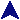Exam II Study GuideCSc 231 Study GuidesMaterial for the second exam will overlap considerably the first exam, most notably in patterns. Here's a list of things which would be good to know:
1. Be able to understand and create patterns. Here are some particular things to concentrate on.
1. Know what the `=~` and `!~` operators do.
2. Know how to create and use pattern matches and pattern replacements.
3. Know how to use at least the following pattern operators: `*`, `+`, `^`, `\$`, `{}`, `[]`, `.`, `?`, `\s` and `\S`.
4. Know how to `^` behaves in and out of `[]`.
5. Know how to use parentheses to capture a portion of a match into `\$`n variables.
6. Know that patterns interpolate variables.
2. References, packages, and modules.
1. Know how to create a reference to an existing variable, and how to use a reference.
2. Know how to pass references as parameters, and how to use them.
3. Know how to create a package, and how to create a module.
4. Know what a blessed reference is, and how to create one.
5. Know how a method call of the form `\$r->f(x)`, for a blessed reference `\$r`, is translated.
6. Know how to create objects in perl.
3. CGI
1. Know how a CGI program differs from a static web page.
2. Know how the user can get data to a CGI script.
1. Through the URL as a query.
2. Through the URL as path info.
3. Through a form.
3. Know how form data is sent to a CGI script.
4. Know the difference between the POST and GET methods for processing forms.
5. Know what the CGI script must generate.
1. Don't forget the content type line.
2. Know why a CGI script cannot just die.
6. Know about the CGI module.
1. Know what sort of decoding it must provide for incoming data.
2. Know how to create a query object.
3. Know how to extract form data from the query object.
4. Know how to use the query object to generate HTML.